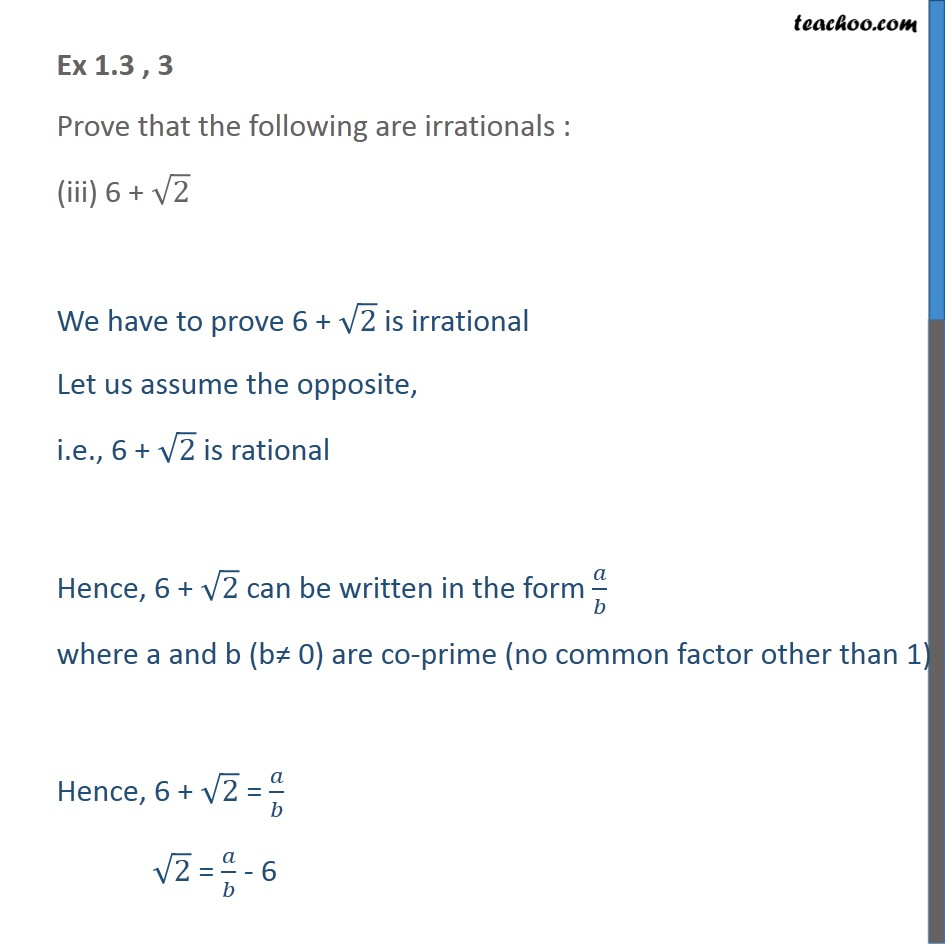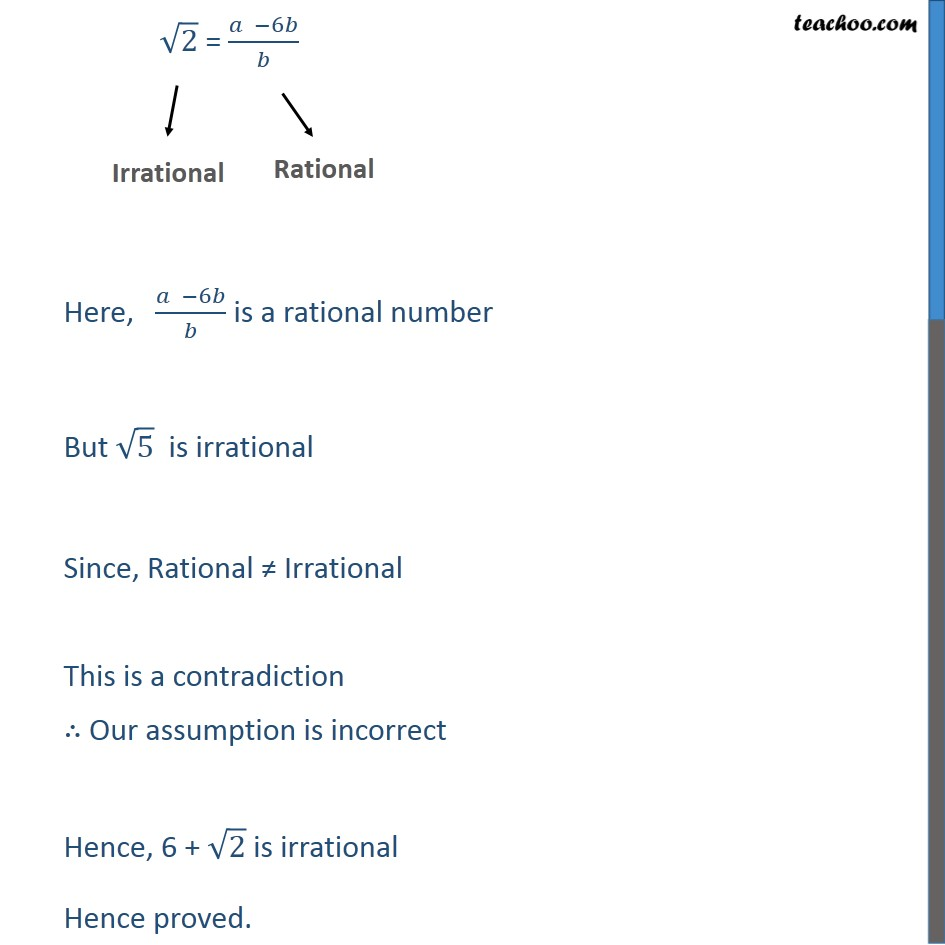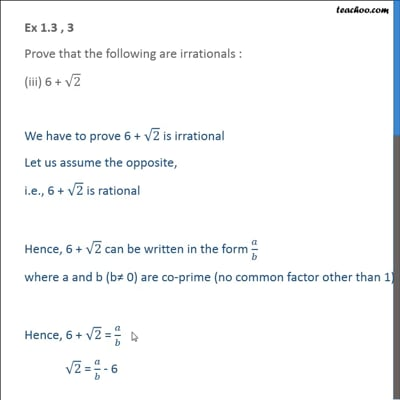Ex 1.3

Chapter 1 Class 10 Real Numbers
Serial order wiseThis video is only available for Teachoo black users

Introducing your new favourite teacher - Teachoo Black, at only ₹83 per month

### Transcript

Ex 1.3 , 3 Prove that the following are irrationals : (iii) 6 + √2 We have to prove 6 + √2 is irrational Let us assume the opposite, i.e., 6 + √2 is rational Hence, 6 + √2 can be written in the form 𝑎/𝑏 where a and b (b≠ 0) are co-prime (no common factor other than 1) Hence, 6 + √2 = 𝑎/𝑏 √2 = 𝑎/𝑏 - 6 √2 = (𝑎 −6𝑏)/𝑏 Here, (𝑎 −6𝑏)/𝑏 is a rational number But √5 is irrational Since, Rational ≠ Irrational This is a contradiction ∴ Our assumption is incorrect Hence, 6 + √2 is irrational Hence proved.